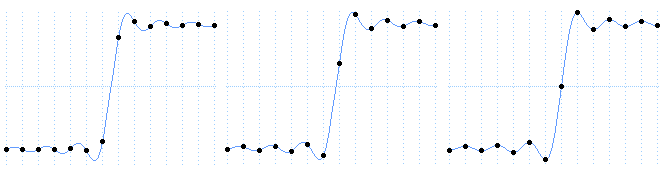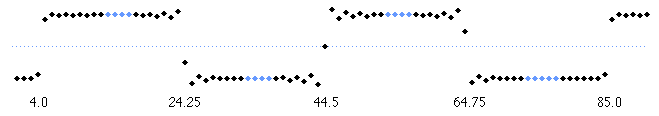# 11. Implementation

Home | Next

Since the same band-limited step will be sampled over and over, it is more efficient to do this ahead of time.

The result of sampling a band-limited step at regular intervals depends on where the exact center falls with respect to sample points; at one point the result has few ripples, and at a half sample in either direction there are many. Thus, several sampled versions of the step will be necessary, each having a slightly different sub-sample offset at which the amplitude transition occurs. Here are three samplings at different subsample offsets:Transitions that occur between two samples, nearer to one sample, and exactly on a sample

The result is a set of sampled steps corresponding to the subsample offset at which the transition occurs:Sampled steps for offsets 0, 0.25, 0.50, and 0.75

The following square wave has transitions every 20.25 samples, at the time points shown. It has been constructed of 16-sample steps (in black). Note how the fraction alone determines which set of samples are used:An actual implementation would use at a finer subsample resolution (1/32 at minimum) and sample a wider section of the band-limited step (16 or more samples).